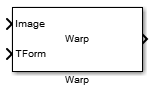# Warp

Apply projective or affine transformation

• Library:
• Computer Vision Toolbox / Geometric Transformations

•## Description

The Warp block transforms an image by applying projective or affine transformation. You can transform the entire image or a region of the image by defining a rectangular region of interest (ROI).

## Ports

### Input

expand all

Input image, specified as one of these values:

• matrix — For intensity images of size M-by-N.

• 3-D numeric array — For true color images of size M-by-N-by-3.

Data Types: `single` | `double` | `int8` | `int16` | `int32` | `int64` | `uint8` | `uint16` | `uint32` | `uint64`

Transformation matrix, specified as any one of these values:

• 3-by-2 matrix — For affine transformation.

• 3-by-3 matrix — For projective transformation.

#### Dependencies

To enable this input port, set the Transformation matrix source parameter value to `Input port`.

Data Types: `double` | `single`

Region of interest, specified as a 4-element vector of form [xs ys width height]. xs and ys are the x and y coordinates of the top left corner of the ROI, respectively.

If you specify the `ROI` input, the `Warp` block applies transformation only to the specified region and returns the transformed region at the output.

#### Dependencies

To enable this input port, select the Enable ROI input port parameter.

### Output

expand all

Transformed image, returned as one of these values:

• matrix — If input is an intensity image of size P-by-Q1.

• 3-D numeric array — If input is a true color image of size P-by-Q-by-3.

The data type of the output transformed image is same as that of the input image. The size of the output transformed image is either same as the input image or equal to the value set for the Output image position vector [x y width height] parameter.

Data Types: `single` | `double` | `int8` | `int16` | `int32` | `int64` | `uint8` | `uint16` | `uint32` | `uint64`

Indicator for transformed ROI outside image region, returned as `0` or `1`.

Data Types: `Boolean`

## Parameters

expand all

Source for passing transformation matrix, specified as either ```Input port``` or `Custom`. If you select `Custom`, you can enter the transformation matrix coefficients by using the Transformation matrix parameter that appears with this selection.

Value for transformation matrix, specified as a 3-by-2 matrix for affine transformation or 3-by-3 matrix for projective transformation.

#### Dependencies

To enable this parameter, set the Transformation matrix for source parameter value to `Custom`.

Method for interpolating transformed pixel values, specified as ```Nearest neighbor```, `Bilinear`, or `Bicubic`.

If you select `Nearest neighbor`, the block uses the value of an nearby pixel for the new pixel value. If you select `Bilinear`, the new pixel value is the weighted average of the four nearest pixel values. If you select `Bicubic`, the new pixel value is the weighted average of the sixteen nearest pixel values.

The number of pixels the block considers affects the complexity of the computation. Therefore, the `Nearest neighbor` interpolation is the most computationally efficient. However, because the accuracy of the method is proportional to the number of pixels considered, the `Bicubic` method is the most accurate. For information about the interpolation methods, see the More About section.

Intensity value for background pixels in the transformed image, specified as one of these values:

• scalar — If input image is a gray scale image.

• 3-element vector — If input image is a true color image. The vector is of the form [r g b] specifying the red (r), green (g), and blue (b) color channel values for the background pixels.

The default fill value is `0` and sets the background color to black.

Source for passing a value for the output image size, specified as either `Same as input image` or `Custom`.

• If you select `Same as input image`, the output transformed image is of same size as that of the input image.

• If you select `Custom`, you must specify a bounding box to output only the image region that lies within the bounding box. This selection enables the Output image position vector [x y width height] parameter that you can use for specifying the bounding box value.

Size of the output image, specified as a four element vector of form [x y width height]. When you specify this parameter, the `Warp` block creates a bounding box of specified width and height values. The size of the output image is set to the size of the bounding box and will contain the transformed image region that lies within the bounding box. If the size of the output image is greater than the size of the transformed image region within the bounding box, the intensity value of the extraneous pixels in the output image are set to the value specified for Background fill value parameter.x and y are the spatial coordinates that define top-left corner position of the bounding box with respect to the input image.

#### Dependencies

To enable this parameter, set the Output image position source parameter value to `Custom`.

Select this parameter to enable the `ROI` input port and specify the ROI to be transformed.

Select this parameter to enable the `Err_roi` output port.

#### Dependencies

To enable this parameter, select the Enable ROI input port parameter.

Block simulation method, specified as `Interpreted Execution` or `Code Generation`. If you want your block to use the MATLAB® interpreter, choose `Interpreted Execution`. If you want your block to run as compiled code, choose `Code Generation`. For more information, see Choosing a Simulation Mode (Simulink).

## Block Characteristics

 Data Types `Boolean` | `double` | `fixed point` | `integer` | `single` Multidimensional Signals `yes` Variable-Size Signals `yes`

expand all

expand all

## Version History

Introduced in R2015b